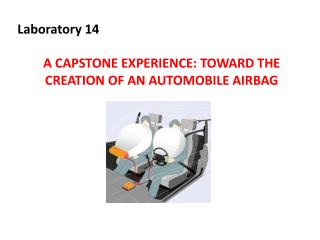DownloadDownload PresentationLaboratory 14 A CAPSTONE EXPERIENCE: TOWARD THE CREATION OF AN AUTOMOBILE AIRBAG

# Laboratory 14 A CAPSTONE EXPERIENCE: TOWARD THE CREATION OF AN AUTOMOBILE AIRBAG

Download Presentation## Laboratory 14 A CAPSTONE EXPERIENCE: TOWARD THE CREATION OF AN AUTOMOBILE AIRBAG

- - - - - - - - - - - - - - - - - - - - - - - - - - - E N D - - - - - - - - - - - - - - - - - - - - - - - - - - -
##### Presentation Transcript

1. Laboratory 14 A CAPSTONE EXPERIENCE: TOWARD THE CREATION OF AN AUTOMOBILE AIRBAG

2. Objectives • Integrate general chemistry I concepts to create a model for an automobile airbag • Determine a method and then carry out appropriate procedures to inflate a bag with a gas, given a specified set of materials

3. THREE STATES OF MATTER General Properties of Gases • There is a lot of “free” space in a gas. • Gases can be expanded infinitely. • Gases fill containers uniformly and completely. • Gases diffuse and mix rapidly.

4. Properties of Gases • Gas properties can be modeled using math. Model depends on— • V = volume of the gas (L) • T = temperature (K) • n = amount (moles) • P = pressure (atmospheres)

5. The Ideal Gas Equation Ideal gas law the functional relationship between the pressure, volume, temperature and moles of a gas. PV = nRT P = pressure V = volume T = temperature (Kelvin) n = number of moles R = gas constant

6. Standard molar volume of an ideal gas The volume of 1 mol of an ideal gas under standard conditions, V = nRT = (1 mol) [0.082057 (L•atm)/(K•mol)] (273.15 K) = 22.41 L P 1 atm The volume of 1 mol of an ideal gas at 0ºC and 1 atm pressure is 22.41 L, called the standard molar volume of an ideal gas.

7. Using PV = nRT How much N2 is required to fill a small room with a volume of 960 cubic feet (27,000 L) to 745 mm Hg at 25 oC?

8. Solution • 1. Get all data into proper units • V = 27,000 L • T = 25 oC + 273 = 298 K • P = 745 mm Hg (1 atm/760 mm Hg) = 0.98 atm • R= 0.0821 L atm / mol K

9. Now plug in those values and solve for the unknown. PV = nRT n = 1.1 x 103 mol (or about 30 kg of gas)

10. Importance of Gases • Airbags fill with N2 gas in an accident. • Gas is generated by the decomposition of sodium azide, NaN3. • 2 NaN3 ---> 2 Na + 3 N2 • Electronic sensors built into the car detect collisions, and an electrical impulse is sent to the air bag, igniting it. • The air bag itself is made of nylon and contains very small pores, and will deflate soon after inflating to allow the passenger to exit the vehicle.

11. Your task is to simulate the construction of an air bag using a ziplock bag, baking soda (NaHCO3) and acetic acid (HC2H3O2) The reaction produces , which inflates the ziplock bag. carbon dioxide gas NaHCO3(s) + HC2H3O2(aq)  (baking soda) (acetic acid ) CO2(g) + NaC2H3O2(aq) + H2O(l)